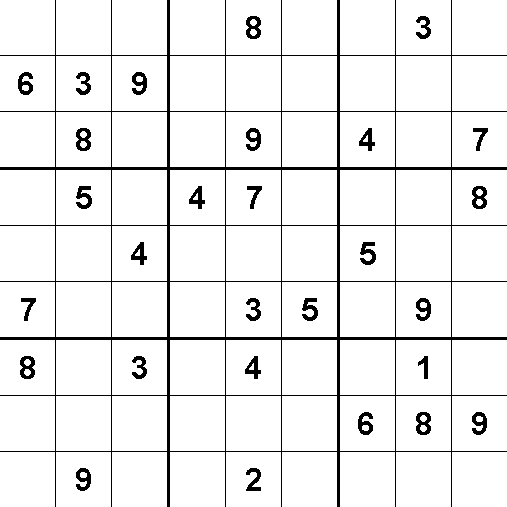# Square Sūdoku

Probability Level 2A sūdoku is a puzzle on a 9x9 grid, in which are numbers from 1 to 9 arranged in a fixed pattern. Each box (a total of 9 are present) contains all the numbers from 1 to 9, and so do each row and column. Some numbers are given, and we are to find the rest. But here you don't need to solve the puzzle, you need to answer a relatively easier question-

How many squares are there in a sūdoku puzzle?

Details- I just noticed that the image is not enclosed by a square, but assume the sūdoku puzzle to be inside a big square.

×